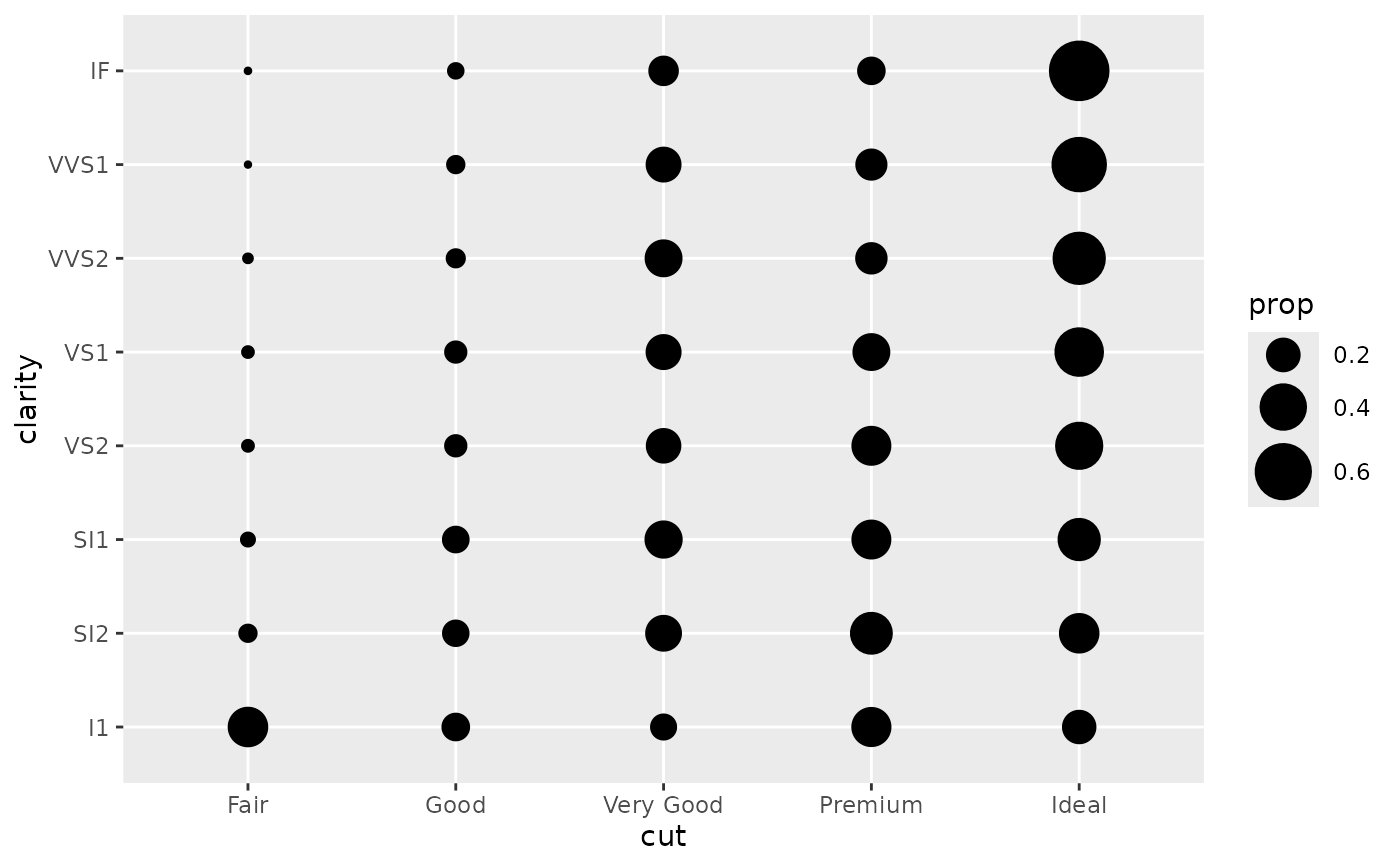This is a variant geom_point() that counts the number of observations at each location, then maps the count to point area. It useful when you have discrete data and overplotting.

## Usage

geom_count(
mapping = NULL,
data = NULL,
stat = "sum",
position = "identity",
...,
na.rm = FALSE,
show.legend = NA,
inherit.aes = TRUE
)

stat_sum(
mapping = NULL,
data = NULL,
geom = "point",
position = "identity",
...,
na.rm = FALSE,
show.legend = NA,
inherit.aes = TRUE
)

## Arguments

mapping

Set of aesthetic mappings created by aes(). If specified and inherit.aes = TRUE (the default), it is combined with the default mapping at the top level of the plot. You must supply mapping if there is no plot mapping.

data

The data to be displayed in this layer. There are three options:

If NULL, the default, the data is inherited from the plot data as specified in the call to ggplot().

A data.frame, or other object, will override the plot data. All objects will be fortified to produce a data frame. See fortify() for which variables will be created.

A function will be called with a single argument, the plot data. The return value must be a data.frame, and will be used as the layer data. A function can be created from a formula (e.g. ~ head(.x, 10)).

position

Position adjustment, either as a string naming the adjustment (e.g. "jitter" to use position_jitter), or the result of a call to a position adjustment function. Use the latter if you need to change the settings of the adjustment.

...

Other arguments passed on to layer(). These are often aesthetics, used to set an aesthetic to a fixed value, like colour = "red" or size = 3. They may also be parameters to the paired geom/stat.

na.rm

If FALSE, the default, missing values are removed with a warning. If TRUE, missing values are silently removed.

show.legend

logical. Should this layer be included in the legends? NA, the default, includes if any aesthetics are mapped. FALSE never includes, and TRUE always includes. It can also be a named logical vector to finely select the aesthetics to display.

inherit.aes

If FALSE, overrides the default aesthetics, rather than combining with them. This is most useful for helper functions that define both data and aesthetics and shouldn't inherit behaviour from the default plot specification, e.g. borders().

geom, stat

Use to override the default connection between geom_count() and stat_sum().

## Aesthetics

geom_point() understands the following aesthetics (required aesthetics are in bold):

• x

• y

• alpha

• colour

• fill

• group

• shape

• size

• stroke

Learn more about setting these aesthetics in vignette("ggplot2-specs").

## Computed variables

These are calculated by the 'stat' part of layers and can be accessed with delayed evaluation.

• after_stat(n)
Number of observations at position.

• after_stat(prop)
Percent of points in that panel at that position.

For continuous x and y, use geom_bin_2d().

## Examples

ggplot(mpg, aes(cty, hwy)) +
geom_point()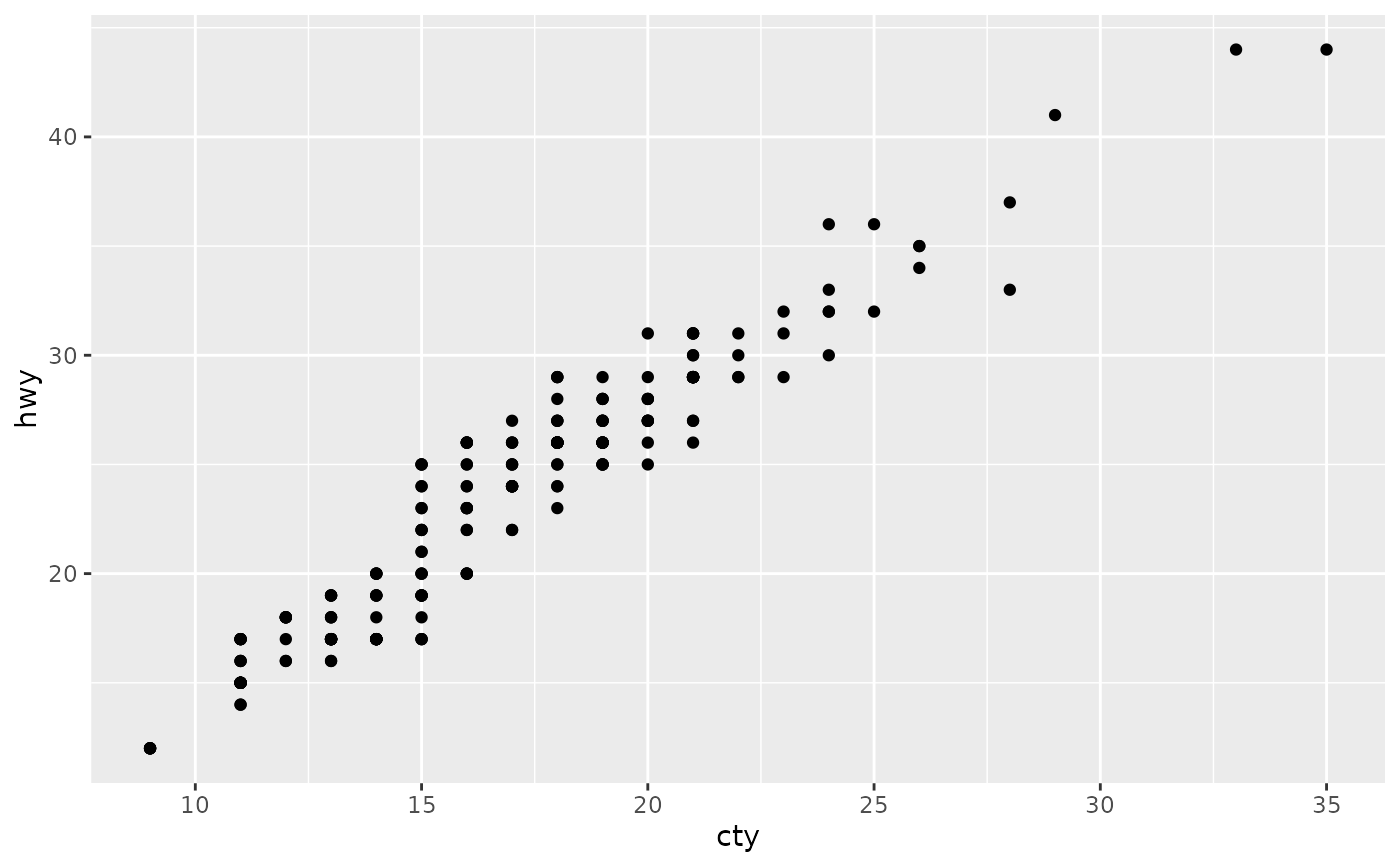ggplot(mpg, aes(cty, hwy)) +
geom_count()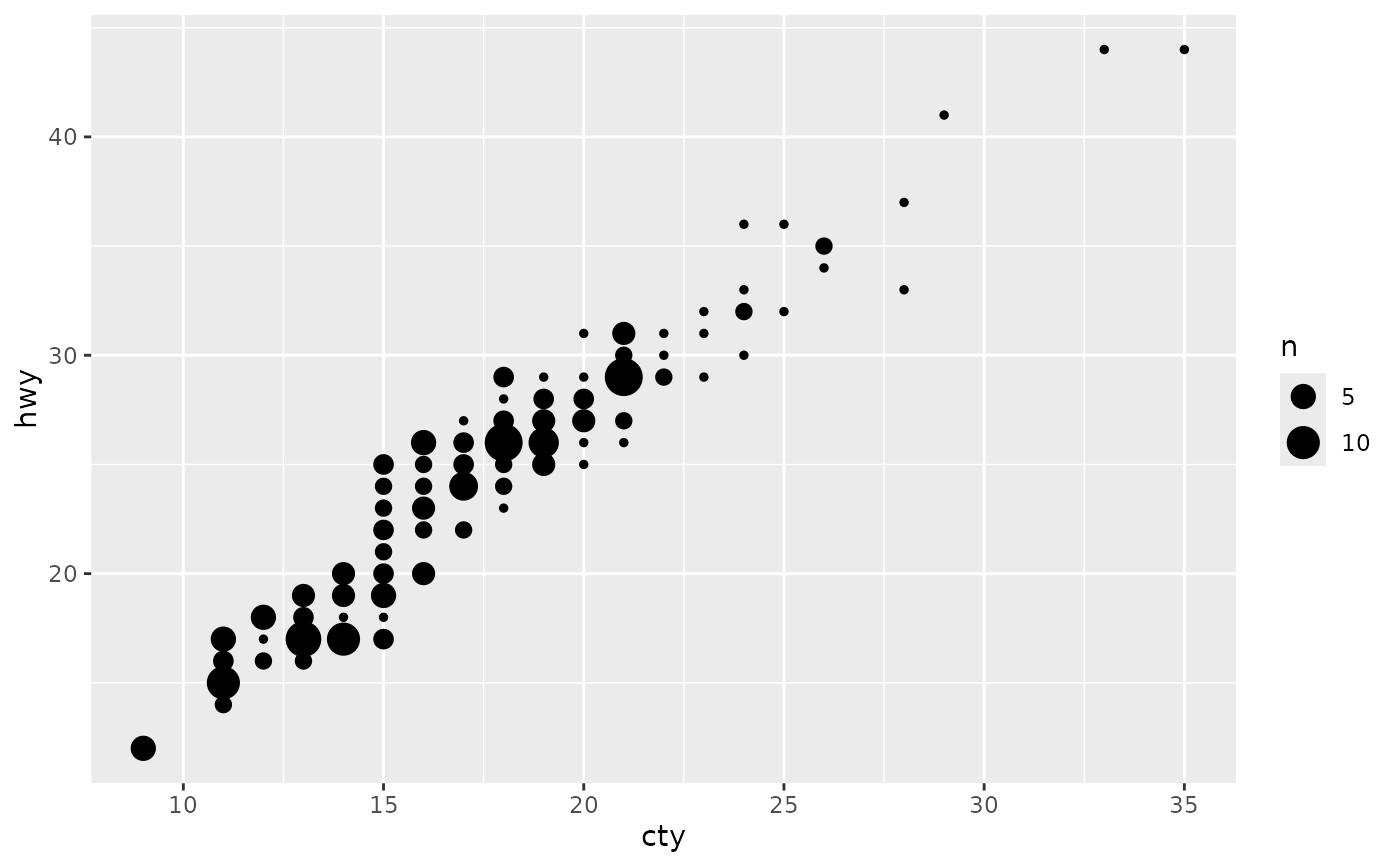# Best used in conjunction with scale_size_area which ensures that
# counts of zero would be given size 0. Doesn't make much different
# here because the smallest count is already close to 0.
ggplot(mpg, aes(cty, hwy)) +
geom_count() +
scale_size_area()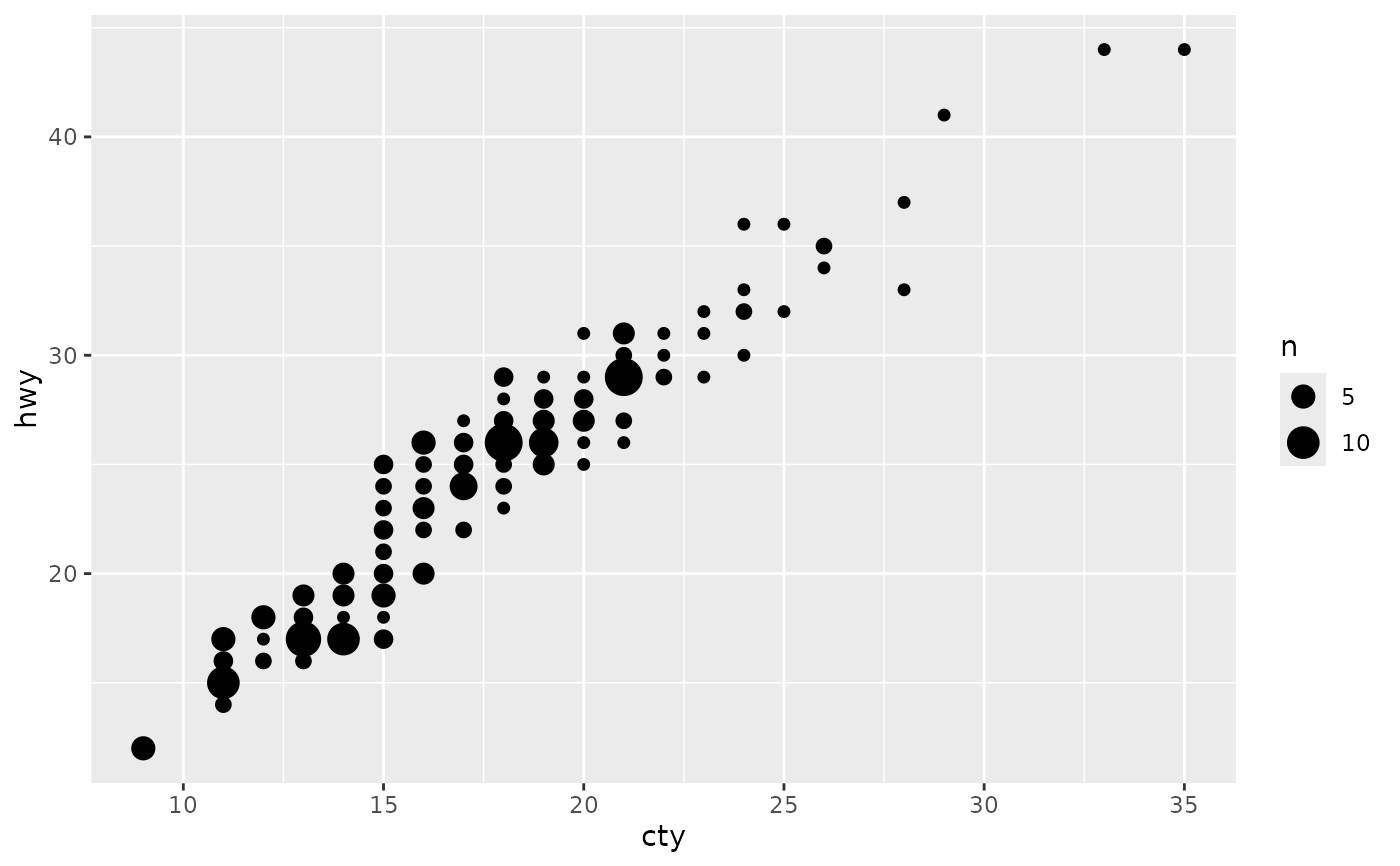# Display proportions instead of counts -------------------------------------
# By default, all categorical variables in the plot form the groups.
# Specifying geom_count without a group identifier leads to a plot which is
# not useful:
d <- ggplot(diamonds, aes(x = cut, y = clarity))
d + geom_count(aes(size = after_stat(prop)))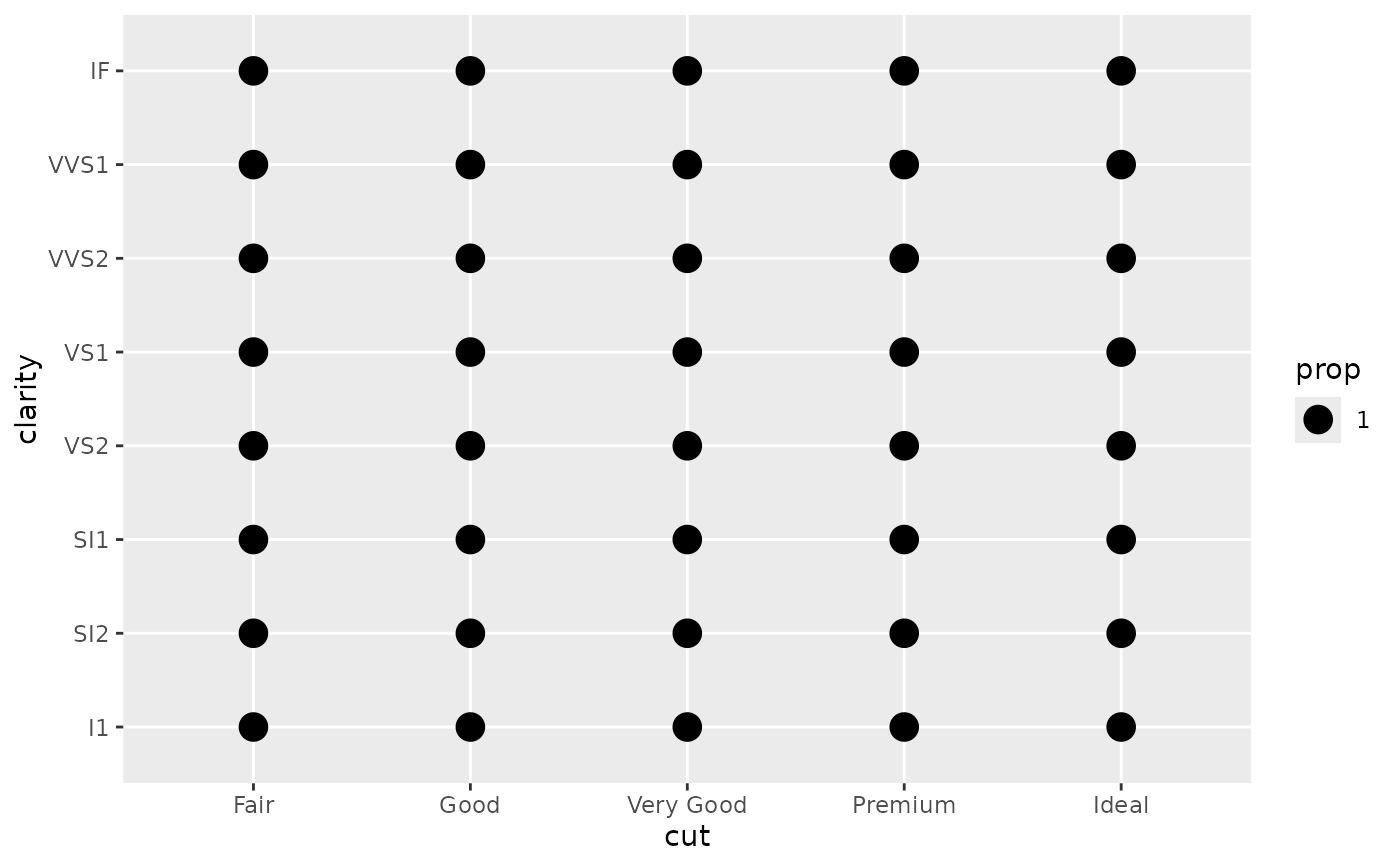# To correct this problem and achieve a more desirable plot, we need
# to specify which group the proportion is to be calculated over.
d + geom_count(aes(size = after_stat(prop), group = 1)) +
scale_size_area(max_size = 10)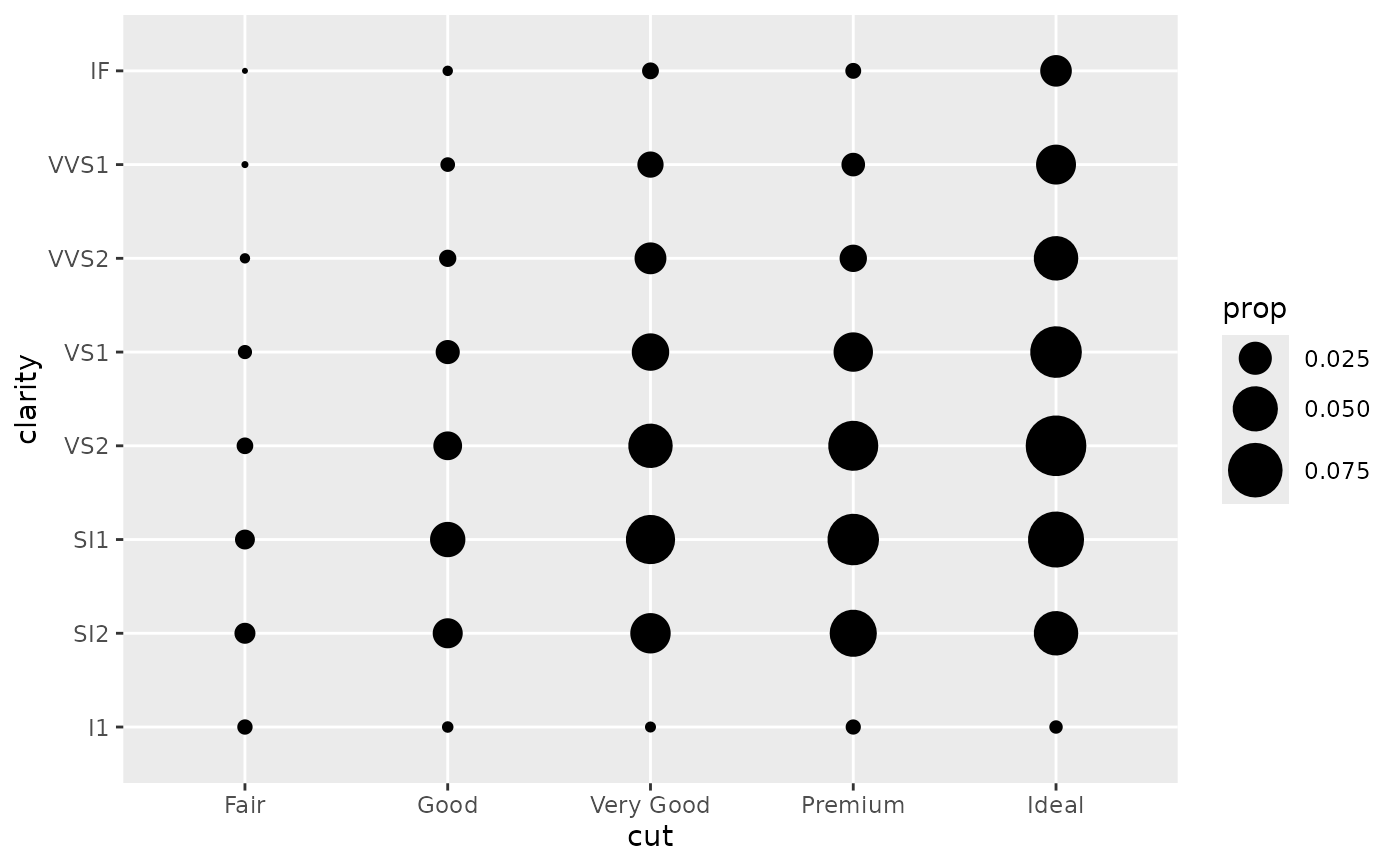# Or group by x/y variables to have rows/columns sum to 1.
d + geom_count(aes(size = after_stat(prop), group = cut)) +
scale_size_area(max_size = 10)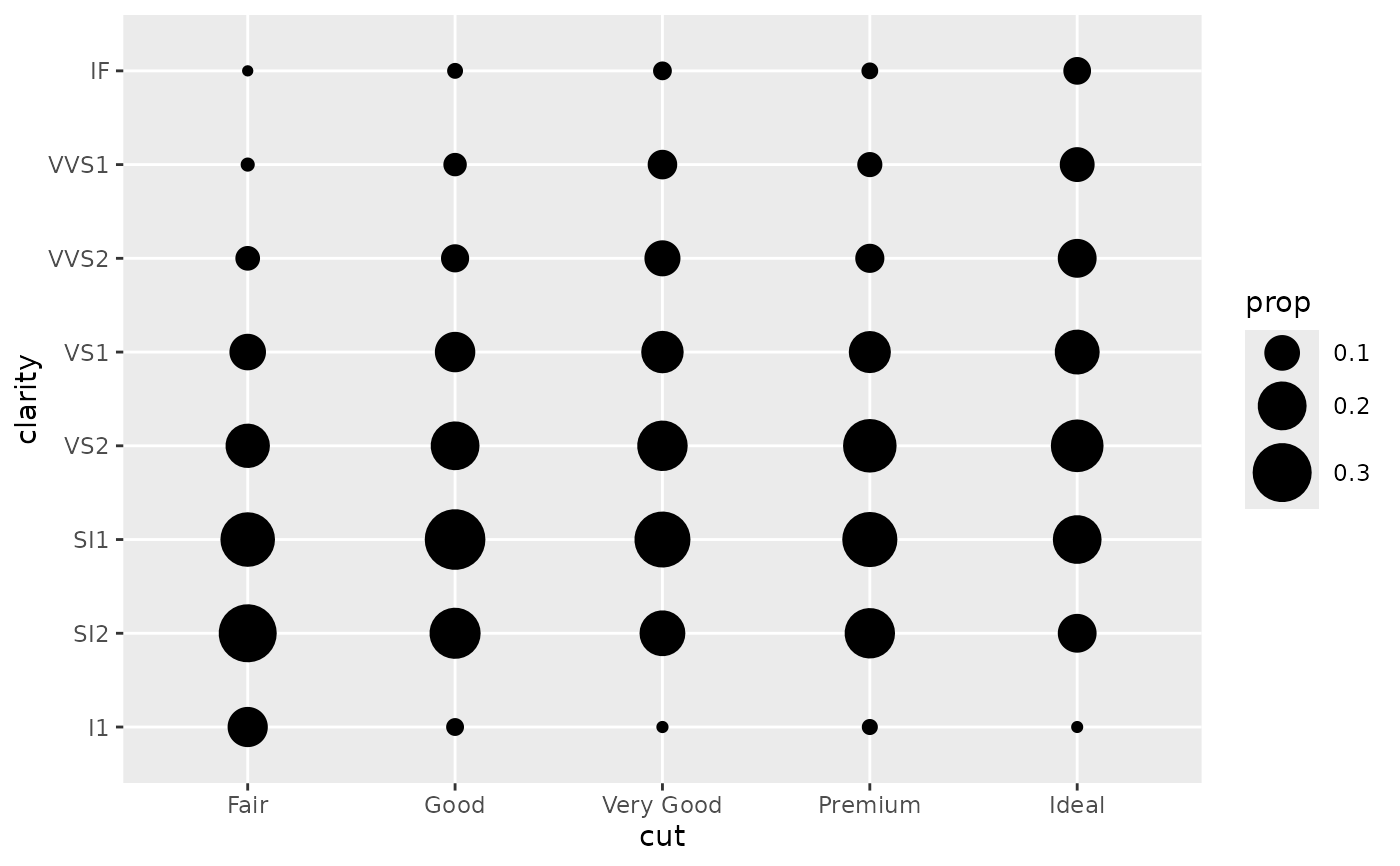d + geom_count(aes(size = after_stat(prop), group = clarity)) +
scale_size_area(max_size = 10)